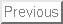Next: Bibliography Up:Back to Publications Page

# THE ASTEROID IDENTIFICATION PROBLEM

M.E. Sansaturio
E.T.S.I.I., Univ. Valladolid; marsan@wmatem.eis.uva.es

Andrea Milani
Dip. Matematica, Università di Pisa; milani@dm.unipi.it

A. La Spina
Dip. Matematica, Università di Pisa; laspina@dm.unipi.it

A large fraction of the asteroids observed so far are to be considered lost, that is they can not be recovered by pointing the telescope at the predicted position only. The catalogues of asteroid orbits are therefore polluted by large numbers of low accuracy orbits, which can not be easily improved by observation. Two of these inaccurate orbits can belong to the same physical object, and indeed identifications are regularly found when the orbital elements are close; an unknown number of real identifications have not been found in the existing catalogues because the orbital elements as computed are far apart. It is impossible to systematically check for possible identification of all the couples of orbital element sets; an algorithm is required to single out a comparatively small number of proposed identifications, and to find a suitable first guess within the region of convergence of the differential corrections procedure to be used to confirm the identification.

In the linear approximation the target function to be minimized is a quadratic form; thus a fully analytic algorithm can be given to compute both the first guess for an identification and the cost, that is the increase in the target function resulting from joining the two sets of observations. This allows to define a number of distances in the elements space, which are functions, not only of the two orbital element sets, but also of their uncertaintices, as measured by the Gaussian covariance matrices. Tests on the values of these distances are used as preliminary filters, to allow more extensive computations to be performed on a fraction of the total number of possible couples.

The difficulties in the application of this algorithm arise from the fact that poorly observed orbits have badly conditioned covariance matrices: all the computations performed with these matrices are affected by large numerical errors. Moreover, it is clear that the larger the eigenvalues of the covariance matrix, the larger the nonlinear effects will be. For asteroids observed over a very short arc, and lost since a long time, the linear approximation fails. Nonlinear optimisation algorithms can be used but are computationally expensive . Identifications based upon some of the orbital elements, e.g., excluding the mean longitude, can be effective in reducing the relevance of the nonlinear effects; the corresponding distances are defined by the marginal covariance matrices, using a formalism developed in .

We propose an orbit identification procedure involving a cascade of filters, using first a distance computed using only the inclination and the node, then a distance using all elements but the mean longitude, and finally a full 6-elements distance. In each stage the distance depends also upon the relevant covariance matrices. We have tested this algorithm on a set of 100 already identified orbits, and found that all of them could have been obtained with a fully automated procedure. We suggest that either our procedure or an equivalent one should be applied as a matter of routine to all newly discovered asteroids. The search for orbit identifications in the existing catalogues can be done in a similar way, but requires to recompute--with the utmost care in guaranteeing numerical accuracy--all the orbits and their normal/covariance matrices, and this in turn requires unrestricted access to the data set of all the astrometric observations.Next: Bibliography Up:Back to Publications Page

1998-10-23